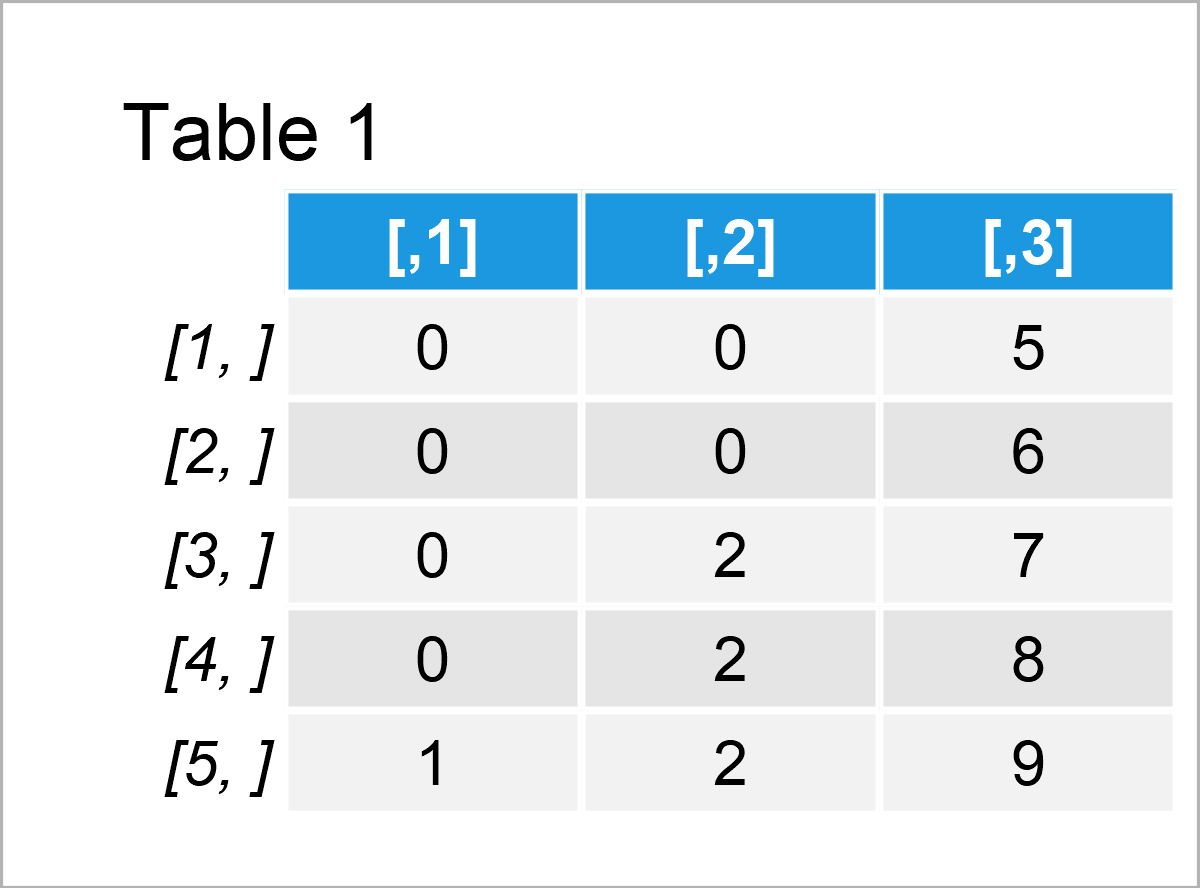# Split Number into Digits in R (2 Examples)

In this article you’ll learn how to convert a number into digits in R.

## Importing the TeachingDemos Package

For the examples of this tutorial, we’ll first have to load the TeachingDemos package:

```install.packages("TeachingDemos")           # Install & load TeachingDemos
library("TeachingDemos")```

## Example 1: Split Single Number into Digits

In this example, I’ll explain how to divide a single number into digits.

Let’s create a data object containing such a number:

```x1 <- 13579                                 # Create single example number
x1                                          # Print example number
#  13579```

Next, we can apply the digits() function of the TeachingDemos package to split our number into digits:

```x1_split1 <- digits(x1)                     # Split number into digits
x1_split1                                   # Print digits output
#  1 3 5 7 9```

We may also use the n argument of the digits function to add several 0 digits in front of the output:

```x1_split2 <- digits(x1, n = 10)             # Specify number of digits
x1_split2                                   # Print digits output
#   0 0 0 0 0 1 3 5 7 9```

## Example 2: Split Vector of Numbers into Digits

The following syntax illustrates how to separate a vector of numbers into digits.

Once again, let’s create some example data:

```x2 <- c(1, 222, 56789)                      # Create vector of numbers
x2                                          # Print vector of numbers
#      1   222 56789```

If we apply the digits function to this vector, a list object is returned. Each of the list elements corresponds to one of the vector elements:

```x2_split1 <- digits(x2)                     # Split vector of numbers
x2_split1                                   # Print digits output
# []
#  1
#
# []
#  2 2 2
#
# []
#  5 6 7 8 9```

We can also use the simplify argument to return a matrix instead of a list:

```x2_split2 <- digits(x2, simplify = TRUE)    # Matrix of digits
x2_split2                                   # Print digits output```Furthermore, we might combine the digits function with the unlist function to convert the output to a vector of digits:

```x2_split3 <- unlist(digits(x2))             # Convert list into vector
x2_split3                                   # Print digits output
#  1 2 2 2 5 6 7 8 9```

## Video, Further Resources & Summary

Do you want to learn more about the splitting of a number into digits using the digits() function of the TeachingDemos package? Then you might watch the following video on my YouTube channel. In the video, I show the contents of this tutorial:

Furthermore, you may want to read the related tutorials on this website:

In summary: In this tutorial, I have illustrated how to convert a number into digits using the digits() function of the TeachingDemos package in the R programming language. Please let me know in the comments, in case you have any additional comments or questions. Furthermore, don’t forget to subscribe to my email newsletter to receive regular updates on the newest articles.

Subscribe to the Statistics Globe Newsletter